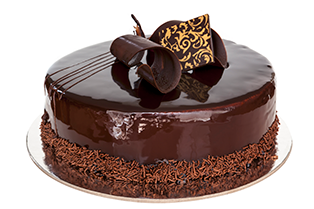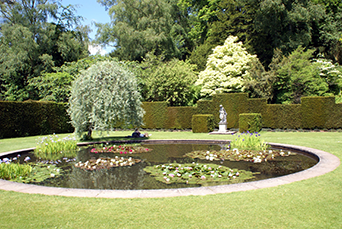Everyday maths 2 (Wales)

Start this free course now. Just create an account and sign in. Enrol and complete the course for a free statement of participation or digital badge if available.

# 1.3 Circumference of a circle

You may have noticed that a new term has slipped in to the title of this section. The term circumference refers to the distance around the outside of a circle – its perimeter. The perimeter and the circumference of a circle mean exactly the same, it’s just that when referring to circles you would normally use the term circumference rather than perimeter.Interactive feature not available in single page view (see it in standard view).

## Activity 3: Finding the circumference

1. You have made a cake and want to decorate it with a ribbon.

The diameter of the cake is 15 cm. You have a length of ribbon that is 0.5 m long. Will you have enough ribbon to go around the outside of the cake?Figure 11 A round chocolate cake
2. You have recently put a pond in your garden and are thinking about putting a fence around it for safety. The radius of the pond is 7.4 m.

What length of fencing would you require to fit around the full length of the pond? Round your answer up to the next full metre.Figure 12 A round garden pond

1. d = 15 cm

Using the formula C = πd

•       C = 3.142 × 15
•       C = 47.13 cm

Since you need 47.13 cm and have ribbon that is 0.5 m (50 cm) long, yes, you have enough ribbon to go around the cake.

2. Radius = 7.4 m so the diameter is 7.4 × 2 = 14.8 m

Using the formula C = πd

C = 3.142 × 14.8

C = 46.5016 m which is 47 m to the next full metre.

You should now be feeling confident with finding the perimeter of all types of shapes, including circles. By completing Activity 3, you have also re-capped on using formulas and rounding.

The next part of this section looks at finding the area (space inside) a shape or space. As mentioned previously, this is incredibly useful in everyday situations such as working out how much carpet or turf to buy, how many rolls of wallpaper you need or how many tins of paint you need to give the wall two coats.

## Summary

In this section you have learned:

• that perimeter is the distance around the outside of a space or shape

• how to find the perimeter of simple and more complex shapes

• how to use the formula for finding the circumference of a circle.#### Find out more

*Eligibility rules apply for financial support.